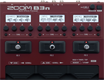# Factory : Gazr

Discussion in 'Zoom B3n' started by Tonelib, May 20, 2018.

1. Factory : GazrDevice: Zoom B3n
Firmware: 2.00

Name on device: Gazr
Optimized for: Phones/Speaker

Effects chain:Huge booming tones, massive reverbs to fill space. Rolling strident tones.

Effect: "Delay" (Delay), active - "yes"
"Time" = 560
"F.B." = 30
"Mix" = 100
"Tail" = Off

Effect: "Plate" (Reverb), active - "yes"
"PreD" = 200
"Decay" = 90
"Mix" = 100
"Tail" = On

Effect: "ReverseDL" (Delay), active - "yes"
"Time" = 1000
"F.B." = 20
"Balance" = 50
"Tail" = Off

Effect: "St Ba GEQ" (Filter), active - "yes"
"50Hz" = 3.0
"120Hz" = 0.0
"400Hz" = -1.0
"500Hz" = -2.5
"800Hz" = -1.5
"4.5kHz" = -0.5
"10kHz" = 5.0
"Volume" = 35
"50Hz" = 10.5
"120Hz" = 0.0
"400Hz" = 0.0
"500Hz" = 0.0
"800Hz" = 0.0
"4.5kHz" = 0.0
"10kHz" = 0.0
"Volume" = 19
"Parameter set" = B

Effect: "Delay" (Delay), active - "yes"
"Time" = 560
"F.B." = 30
"Mix" = 42
"Tail" = Off

Patch Volume: 100